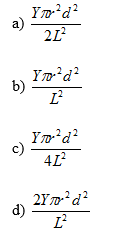Important questions on Elasticity for JEE Main/Advanced and CBSE

In this page we have Important questions on Elasticity for JEE Main/Advanced and CBSE . Hope you like them and do not forget to like , social share and comment at the end of the page.

Multiple Choice questions

Question -1 A wire whose cross-section area is A1 is stretched by L1 by a certain weight. How far will a wire of same material and same length and cross-section area A2 stretch if same weight is applied to it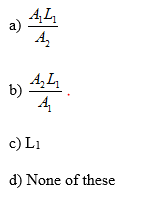Question 2:
A wire is stretched by x mm when a load F is hanged on it.If the same wire goes over a pulley  and two weight F each are hung at the two end’s the what will be the elongation  in the wire
a) x
b) 2x
c) x/2
d) 0

One end of a uniform wire of length L and μ mass per unit length is attached rigidly to a point in a ceiling. A Mass M is suspended from its lower end. Area of cross-section of the wire is A.
Question 3 Find the stress at a distance x from the ceiling point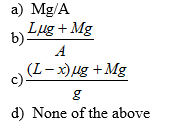Question 4:
Find the elongation of the wire. If Young Modulus of wire is Y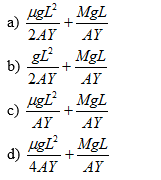Multiple Choice questions
Question 5:  A block of gelatin is 60 mm by 60 mm by 20 mm when unstressed. A force of .245 N is applied tangentially to the upper surface causing a 5mm displacement relative to the lower surface. The block is placed such that 60X60 comes on the lower and upper surface. Find the shearing stress, shearing strain and shear modulus
a) (68.1 N/m2,.25,272.4 N/m2)
b) (68 N/m2,.25,272 N/m2)
c) (67 N/m2,.26,270.4 N/m2)
d) (68.5 N/m2,.27,272.4 N/m2)

Question 6
A wire of radius r is stretched  without sag and tension between two point separated by distance 2L.A weight  is hanged at the middle of the wire which displaced the point by a distance d. Young modulus of elasticity is Y. Find the tension in the wire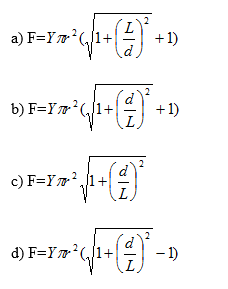Question 7:
If in previous question d<<<<L, what will be the value of Tension